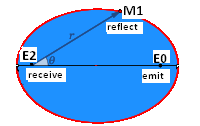# How explain this perturbing equation about the 43 arcseconds?

The planetary orbits have been studied as ellipses but the solar system is in motion in relation to the distant stars. Their path is along the tip of an helix and the ecliptic plane is a convenient plane of projection. I think that the studies were never conducted under this viewpoint.

The sunlight we see now was emitted more than 8 minutes ago when the Sun was ‘below’ the ecliptic but we see it centered in the plane. I wonder why we do not see any consequence of this.

I’m following a line of reasoning that the motion can have consequences and I revisited the anomalous precession of the perihelion of Mercury, settled long time ago by Einstein, and I found this perturbing equation:

$$\frac{43}{5557} = 2\pi\frac{369.2\ \text{km}\ \text{s}^{−1}}{299792.458\ \text{km}\ \text{s}^{−1}}$$

Where 5557 is the predicted theoretical value (in mathpages) for the advance and the 43 is the anomaly.

The simplicity of the formula $$\text{error}/\text{theoric}=2\pi\ V/c$$ and because $V$ is 0.054% off the central measured value of the speed of solar system - $369(\pm0.9$) - makes me wonder if this can be more than a coincidence.

Any kind of reasoning on the why's will be helpful.

The 5557 for the expected precession of Mercury includes 5025 arising from Earth's precession. As I understand it, the true expected (excluding GR) precession of Mercury, ie relative to the fixed stars, is 532. I think that would be a better denominator in your formula.

Also if you applied your approach to Venus, it would give a GR effect similar to that for Mercury, which does not agree with observation.

• As implyed in my own answer (I'm hoping that my reasoning is clearly stated) the effect is due to an error in the measure procedure, thus the total observer-object value is the one that must be considered, imo. The true gravitational distance to Mercury is larger than the one attributed along the ecliptic. The net effect of GR gives an equivalent larger effective radius. – Helder Velez May 25 '14 at 10:20

IMO, the relation may be justified by the way we make measures, a la Einstein: a mean value of a two-way ligth ray path procedure, that is not used in any natural process. This is a human invention.

To illustrate the error in our measures of distance I will make clear the distinction between ‘gravitational distance’ and the ‘Einstein measure’ with the Earth-Moon system with the help of this image.

At the space position $E_0$, and time $t_0$, a laser light is emitted, that propagates with spherical waves in the medium at $c_0$ speed. It is reflected at $M_1$ (Moon’s position at time $t_1$) and, because the Earth is in motion, is received back at position $E_2$ and time $t_2$.
The solar system, hence the Earth and the Moon, is in motion in the direction $\overline{E_0 E_2}$ . The Einstein distance measure is a mean value $d_E= c\cdot (t_2-t_0)/2$ and it reports the same distance when the Moon is located at any point in the red curve at moment $t_1$ (a notable property of ellipses).
At the moment of reception the Moon is at the gravitational distance $r= \overline{E_2 M_1}$ and this is the value that must be used in the inverse square law. The factor of conversion between the Einstein measure $d_E$ and the effective gravitational distance $d_g$ is dependent on the angle of the light ray at reception $\theta$ and obeys the relation $\frac{1-e^2}{1-e\cos\theta}$ where $e$ is the eccentricity. $d_E$ is equal to $d_g$ only for $e=0$, i.e. $V=0$ . This error in the distance measure, or light speed, must have consequences.
I'm not taking in account the light aberration nor the clock rate correction.
Measuring correctly distances is fulcral in energy balance, orbit determination (flyby/pioneers).

Can it be the case that we have bad distances or bad speed of light measures?

! downvote iznogud without a critique, or post an answer !